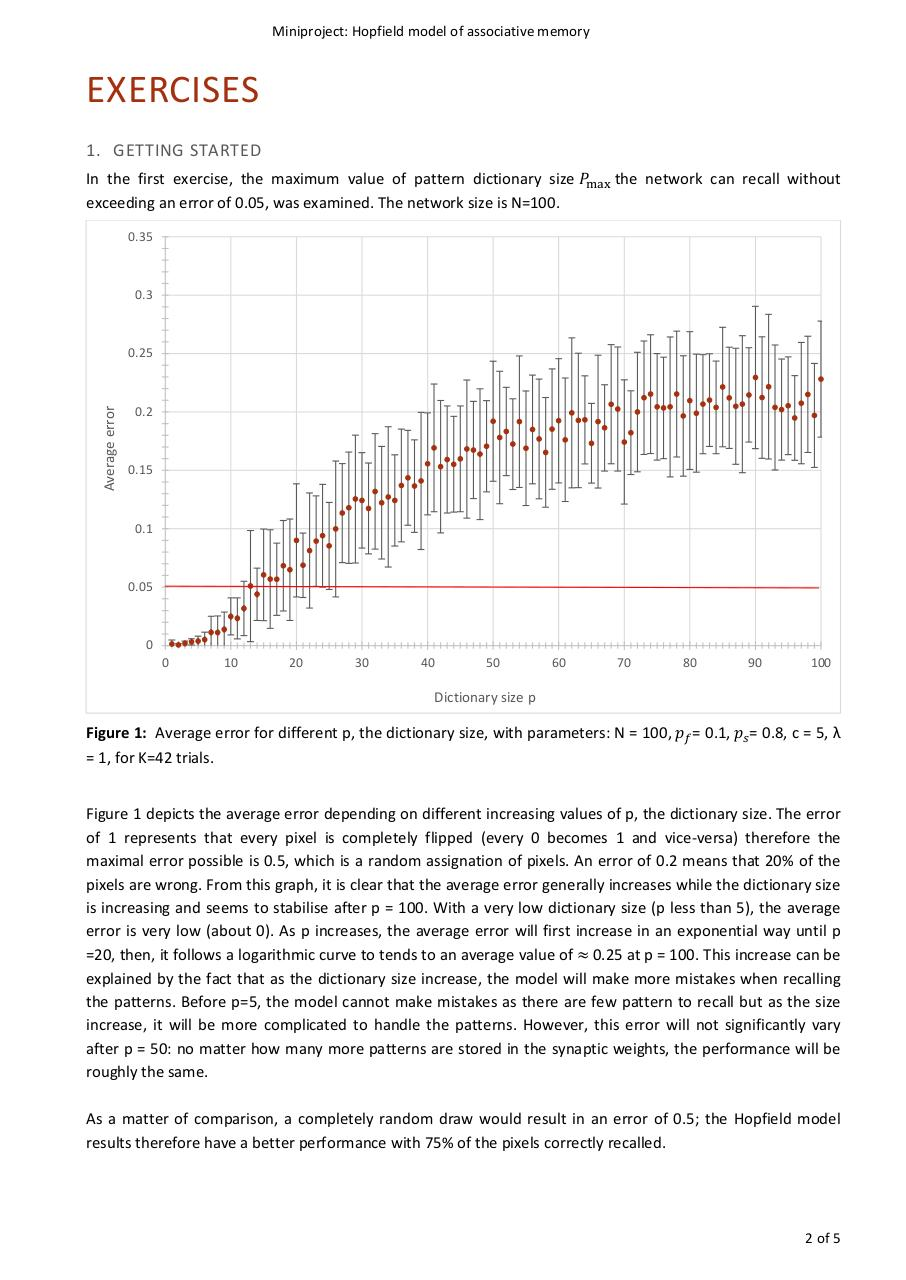# Projet Hopfield.pdfPage 1 2 3 4 5 6

#### Text preview

Miniproject: Hopfield model of associative memory

EXERCISES
1. GETTING STARTED
In the first exercise, the maximum value of pattern dictionary size 𝑃𝑃max the network can recall without
exceeding an error of 0.05, was examined. The network size is N=100.
0.35

0.3

Average error

0.25

0.2

0.15

0.1

0.05

0
0

10

20

30

40

50

60

70

80

90

100

Dictionary size p

Figure 1: Average error for different p, the dictionary size, with parameters: N = 100, 𝑝𝑝𝑓𝑓 = 0.1, 𝑝𝑝𝑠𝑠 = 0.8, c = 5, λ
= 1, for K=42 trials.
Figure 1 depicts the average error depending on different increasing values of p, the dictionary size. The error
of 1 represents that every pixel is completely flipped (every 0 becomes 1 and vice-versa) therefore the
maximal error possible is 0.5, which is a random assignation of pixels. An error of 0.2 means that 20% of the
pixels are wrong. From this graph, it is clear that the average error generally increases while the dictionary size
is increasing and seems to stabilise after p = 100. With a very low dictionary size (p less than 5), the average
error is very low (about 0). As p increases, the average error will first increase in an exponential way until p
=20, then, it follows a logarithmic curve to tends to an average value of ≈ 0.25 at p = 100. This increase can be
explained by the fact that as the dictionary size increase, the model will make more mistakes when recalling
the patterns. Before p=5, the model cannot make mistakes as there are few pattern to recall but as the size
increase, it will be more complicated to handle the patterns. However, this error will not significantly vary
after p = 50: no matter how many more patterns are stored in the synaptic weights, the performance will be
roughly the same.
As a matter of comparison, a completely random draw would result in an error of 0.5; the Hopfield model
results therefore have a better performance with 75% of the pixels correctly recalled.

2 of 5